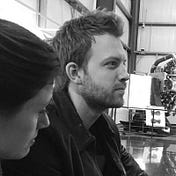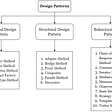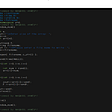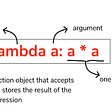# Project Euler: Problem 9

The original problem can be found here

## It states:

`A Pythagorean triplet is a set of three natural numbers, a < b < c, for which a² + b² = c²For example, 32 + 42 = 9 + 16 = 25 = 52.There exists exactly one Pythagorean triplet for which a + b + c = 1000.Find the product abc.`

My friend Anil and I discussed this one last night and I think overcomplicated it a bit, bringing up all sorts of other unmentioned properties of Pythagorean triplets that might be utilized.

However, what I did ended up being pretty simple and it worked very efficiently.

Two facts I based my solution on:

1. Due to the triangle inequality theorem (no one side can be bigger than the sum of the other two) I knew that no side could be bigger than 500 without causing the sum of the three sides to exceed 1000.
2. We have three variables (a, b, c) but only two equations (a² + b² = c², and a+b+c=1000. That’s not enough to solve for any one variable, but it IS enough for us to express the problem as a single expression with two variables.

If you isolate c and reduce to a single expression of a and b, you get:

1000-a-b = sqrt(a² + b²)

All you need to do now is try out all the combinations of a and b and find the one for which that expression is true.

I know that neither a nor b can b greater than 500, so I just filled two arrays with numbers 1 to 500 and cycled them through a nested for loop until I found the pair that satisfied my equation.

## Code:

`#c = sqrt(a**2 + b**2)#abc = a*b*sqrt((a**2) + (b**2)#c = 1000 — a — b# 1000 — a — b = sqrt(a**2 + b**2)import mathx=[]y=[]for i in range(1,501): x.append(i) y.append(i)for a in x: for b in y: if 1000 — a — b == math.sqrt(a**2 + b**2): print “Triplet: “,a,b,(1000-a-b) print a*b*(1000-a-b)`

• The triplet itself is a=375, b=200, and c=425
• The product abc=31,875,000

If you have any suggestions on how I could have improved my algorithm please let me know in the comments!

--

--

--

## More from Lyman Johnson

Django Developer | NCEES-Licensed Engineer in New York, California, and North Carolina

Love podcasts or audiobooks? Learn on the go with our new app.## Lyman Johnson

Django Developer | NCEES-Licensed Engineer in New York, California, and North Carolina

## Python Design Patterns## THE SORTING AESTHETICS.## What is Lambda Function in Python